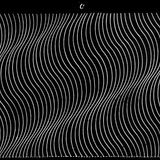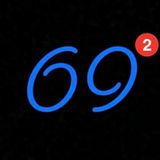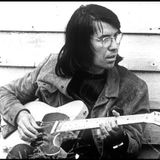# Shows# 47

## by (((sounds)))(((abound)))

1:24:47# 46

## by (((sounds)))(((abound)))

1:14:02# 45

## by (((sounds)))(((abound)))

53:47# 44

## by (((sounds)))(((abound)))

53:50# 43

## by (((sounds)))(((abound)))

36:49# 42

## by (((sounds)))(((abound)))

1:02:46# 41

## by (((sounds)))(((abound)))

1:10:00# 40

## by (((sounds)))(((abound)))

38:06# 39

## by (((sounds)))(((abound)))

50:161:16:44